# Standing waves – problems and solutions

1. The 3-m string tied at one end and the other end is connected to the vibrator. When the vibrator is vibrated, the string formed a stationary wave, as shown in the figure below.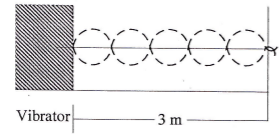Determine the position of the 5th antinode from the fixed end.

Solution :

Distance between two nodes = 3 meters / 5 = 3/5 meters.

The distance between the first node and the fixed end = 3/5 meters

The distance between the second node and the fixed end = 2 (3/5 meters) = 6/5 meters

The distance between the third node and the fixed end = 3 (3/5 meters) = 9/5 meters

The distance between the fourth node and the fixed end = 4 (3/5 meters) = 12/5 meters

Distance between node and antinode = 1/2 (3/5 meters) = 3/10 meters.

Distance between the fifth antinode and the fixed end = distance between the fourth node and the fixed end + distance between node and antinode = 12/5 + 3/10 = 24/10 + 3/10 = 27/10 = 2.7 meters.

[irp]

2. As shown in the figure below, one end connected to the vibrator and another end is fixed. If string’s length is 1.5 meters, find the distance between the fourth node and vibrator.

Solution :

Distance between two nodes = 1.5 meters / 11 = 1.5 / 11 meters.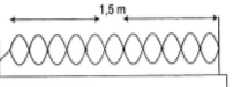Distance between the first node and vibrator = 1.5 / 11 meters

Distance between the second node and vibrator = 2 (1.5 / 11 meters) = 3/11 meters

Distance between the third node and vibrator = 3 (1.5 / 11 meters) = 4.5 / 11 meters

Distance between the fourth node and vibrator = 4 (1.5 / 11 meters) = 6/11 meters = 0.54 meters

[irp]

3. A string has both ends kept fixed, produces a fundamental tone with a frequency of 420 Hz. Determine the third overtone.

A. 840 Hz

B. 1260 Hz

C. 1680 Hz

D. 2940 Hz

Known :

The fundamental frequency (f1) = 420 Hz

Both ends are kept fixed.

Wanted : the third overtone

Solution :

First overtone (f2) = 2 f1 = 2 (420 Hz) = 840 Hz

Second overtone (f3) = 3 f1 = 3 (420 Hz) = 1260 Hz

Third overtone (f4) = 4 f1 = 4 (420 Hz) = 1680 Hz

[irp]

4. The wavelength of the first overtone of a string is 40 cm. If the speed of sound wave in air is 340 m/s, determine the third overtone.

A. 850 Hz

B. 1600 Hz

C. 1700 Hz

D. 3200 Hz

Known :

Wavelength of the first overtone (λ) = 40 cm = 0.4 meters

The speed of the sound wave in air (v) = 340 meters/second

Wanted: frequency of the third overtone

Solution :

Below is a figure of a standing wave on a string with both ends is kept fixed. First, calculate the length of the string using the wavelength of the first overtone. After that, before calculating the frequency of the third overtone, first, calculate the wavelength of the third overtone.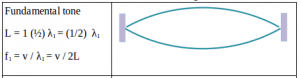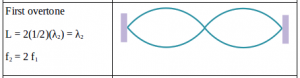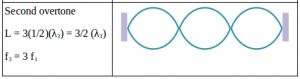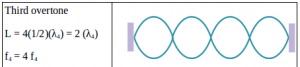Wavelength of the first overtone :

Length of string (L) = 2. ½ λ

Length of string (L) = λ

Length of string (L) = 0.4 meters

Wavelength of the third overtone :

L = 2 λ

0.4 = 2 λ

λ = 0.4 / 2

λ = 0.2 meters

Frequency of the third overtone :

f = v / λ

f = 340 : 0.2

f = 1700 Hertz

[irp]

5. A tube open at both ends with length of 40 cm produces a fundamental tone with frequency of 420 Hz. Determine the second overtone.

A. 380 Hz

B. 460 Hz

C. 840 Hz

D. 1260 Hz

Known :

Length of pipe (L) = 40 cm = 0.4 meters

Frequency of the fundamental tone (f1) = 420 Hertz

Wanted: Frequency of the second overtone (f3)

Solution :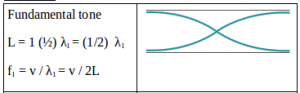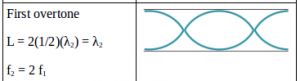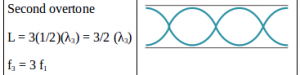If fundamental tone (f1) = 420 Hertz then the second overtone (f3) = 3 f1 = 3 (420 Hertz) = 1260 Hertz

[irp]

6. Sound wave at closed tube has a wave pattern similar to…

A. Wave propagation on a string

B. Wave propagation on-the air column

C. Standing wave on a string fixed at one end

D. Standing wave on a string fixed at both ends

Solution :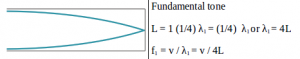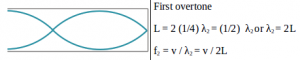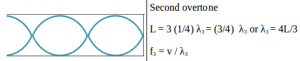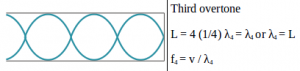A closed tube is a tube that is open at one end but closed at other, as shown in the figure above.